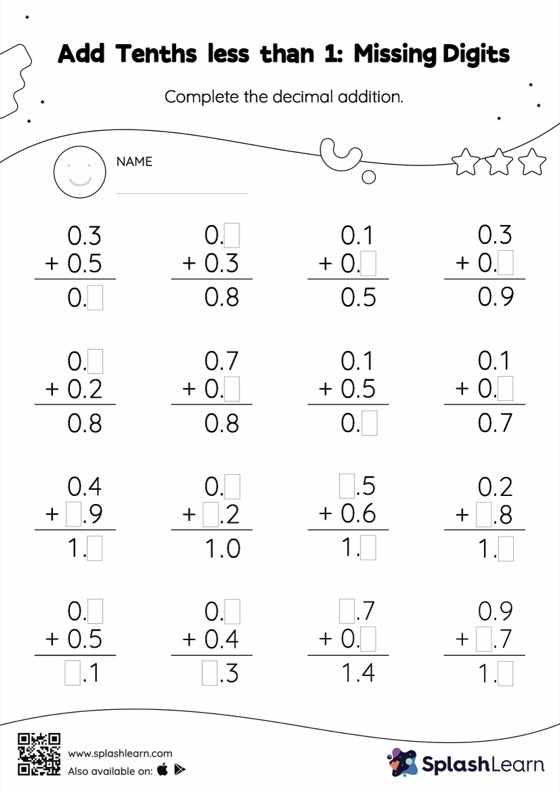# Add Tenths less than 1: Missing Digits Worksheet

Home > Add Tenths less than 1: Missing DigitsWhen adding decimals on this worksheet, students align the decimal points and use zero as a placeholder. Then to find the missing number in the add tenths less than 1 worksheet, students then apply the relationship between addition and subtraction. This worksheet is about practicing the column method in which numbers are written one on top of another. In this method, students use their place value understanding to solve the problems.